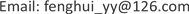Vague集，重点保卫目标，优选与排序，相离度, Vague Set Important Defending Target Selecting and Sequencing Deviation Degree1. 引言

2. Vague集基本理论

Gau和Buehrer早在1993年就提出了Vague集理论  ，由于Vague集理论比较符合人类思维活动，被广泛地推广应用。Vague集理论有以下定义：

3. 防空重点保卫目标综合评价指标体系

4. 基于Vague集的防空重点保卫目标优选与排序方法4.1. 评价指标的模糊值表示

t i j = ( x i j − x j min ) / ( x j max − x j min ) (1)

f i j = ( x j max − x i j ) / ( x j max − x j min ) (2)

t i j = ( x j max − x i j ) / ( x j max − x j min ) (3)

f i j = ( x i j − x j min ) / ( x j max − x j min ) (4)

F i j = t i j + ( 1 − t i j − f i j ) t i j / ( t i j + f i j ) = t i j / ( t i j + f i j ) (5)

4.2. 评价指标权重的计算

J ( X i , X * ) = ∑ j = 1 5 f i j 2 + ( 1 − t i j ) 2 ⋅ w j (8)

m i n   J ( w ) = ∑ i = 1 m ∑ j = 1 5 [ f i j 2 + ( 1 − t i j ) 2 ] w j 2 s . t .   w j ≥ 0 ,   ∑ j = 1 5 w j = 1 (9)

w j = 1 / ∑ j = 1 5 1 ∑ i = 1 m [ f i j 2 + ( 1 − t i j ) 2 ] ∑ i = 1 m [ f i j 2 + ( 1 − t i j ) 2 ] ,   j = 1 , 2 , 3 , 4 (10)

4.3. 基于Vague集的防空重点保卫目标优选方法

1) 构造模糊值矩阵 F = ( F i j ) m × n 。

2) 计算各指标权重。

3) 分别确定方案集的最优、最劣点 X + 、 X − 。

4) 计算每个方案到最优点 X + 的接近度 E i 。

d i + = d ( X i , X + ) = ∑ j = 1 4 ( f i j − f p j ) 2 + ( t i j − t p j ) 2 ⋅ w j ,   i = 1 , 2 , ⋯ , m (11)

d i − = d ( X i , X − ) = ∑ j = 1 4 ( f i j − f q j ) 2 + ( t i j − t q j ) 2 ⋅ w j ,   i = 1 , 2 , ⋯ , m (12)

E i = d i − / ( d i − + d i + ) ,   i = 1 , 2 , ⋯ , m (13)

5) 通过 E i 值对待评价的防空重点保卫目标进行优选与排序。

5. 实例分析

Evaluation indexes of important defending targets in defense zon
U1 U2U3U4
X1 8.257.845.454.60
X29.305.058.267.10
X39.124.406.506.64
X49.085.605.007.06

1) 评价指标模糊值表示

X 1 = { ( U 1 ,   [ 0.00 , 0.00 ] ) ,   ( U 2 ,   [ 1.00 , 1.00 ] ) ,   ( U 3 ,   [ 0.14 , 0.14 ] ) ,   ( U 4 ,   [ 1.00 , 1.00 ] ) }

X 2 = { ( U 1 ,   [ 1.00 , 1.00 ] ) ,   ( U 2 ,   [ 0.19 , 0.19 ] ) ,   ( U 3 ,   [ 1.00 , 1.00 ] ) ,   ( U 4 ,   [ 0.00 , 0.00 ] ) }

X 3 = { ( U 1 ,   [ 0.83 , 0.83 ] ) ,   ( U 2 ,   [ 0.00 , 0.00 ] ) ,   ( U 3 ,   [ 0.46 , 0.46 ] ) ,   ( U 4 ,   [ 0.18 , 0.18 ] ) }

X 4 = { ( U 1 ,   [ 0.79 , 0.79 ] ) ,   ( U 2 ,   [ 0.35 , 0.35 ] ) ,   ( U 3 ,   [ 0.00 , 0.00 ] ) ,   ( U 4 ,   [ 0.02 , 0.02 ] ) }

2) 计算各指标权重分别为： w 1 = 0.4078 ， w 2 = 0.2106 ， w 3 = 0.2153 ， w 4 = 0.1663 。

3) 确定方案集的最优、最劣点 X + 、 X − 。

X + = { ( U 1 ,   [ 1.00 , 1.00 ] ) ,   ( U 2 ,   [ 1.00 , 1.00 ] ) ,   ( U 3 ,   [ 1.00 , 1.00 ] ) ,   ( U 4 ,   [ 1.00 , 1.00 ] ) }

X − = { ( U 1 ,   [ 0.00 , 0.00 ] ) ,   ( U 2 ,   [ 0.00 , 0.00 ] ) ,   ( U 3 ,   [ 0.00 , 0.00 ] ) ,   ( U 4 ,   [ 0.00 , 0.00 ] ) }

4) 确定各方案 X i 到最优、最劣点的距离 d i + 、 d i − 。

d 1 + = 0.7953 ， d 2 + = 0.4807 ， d 3 + = 0.7607 ； d 4 + = 0.8496

d 1 − = 0.5756 ， d 2 − = 0.9377 ， d 3 − = 0.6611 ； d 4 − = 0.5645

5) 确定各方案 X i 到最优点 X + 的接近度 E i 。

E 1 = 0.4199 ， E 2 = 0.6611 ， E 3 = 0.4650 ； E 4 = 0.3992

6. 结束语